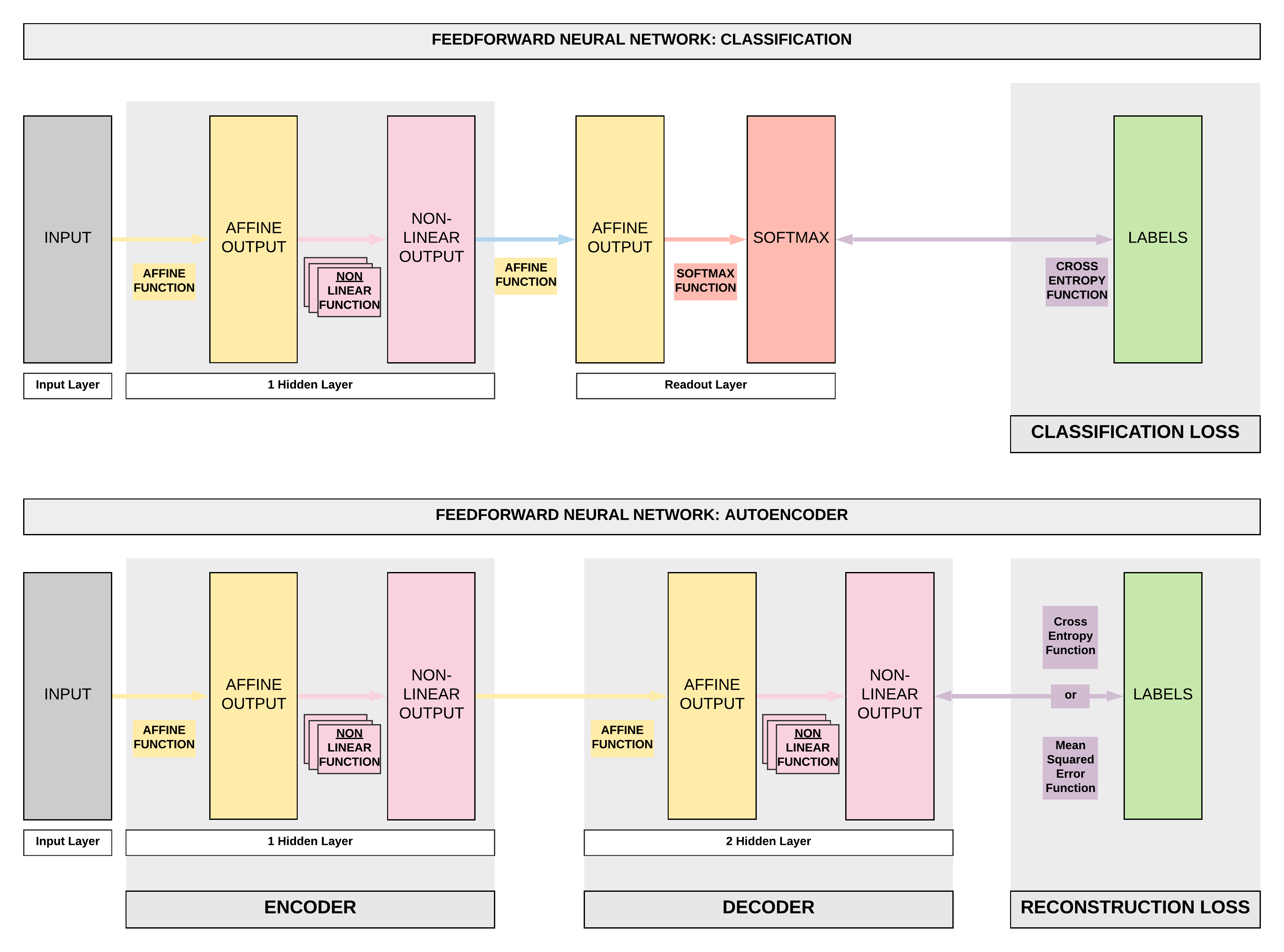# Autoencoders with PyTorch¶

Run Jupyter Notebook

You can run the code for this section in this jupyter notebook link.

### Feedforward Neural Network (FNN) to Autoencoders (AEs)¶

• Autoencoder is a form of unsupervised learning.
• This is a big deviation from what we have been doing: classification and regression which are under supervised learning.
• There are no labels required, inputs are used as labels.
• This is a bit mind-boggling for some, but there're many conrete use cases as you'll soon realize.
• Just a quick preview of use cases we will be covering:
• Denoising overcomplete AEs: recreate images without the random noises originally injected.
• Undercomplete AEs for anomaly detection: use AEs for credit card fraud detection via anomaly detection.
• Variational AEs for creating synthetic faces: with a convolutional VAEs, we can make fake faces.
• An autoencoder's purpose is to learn an approximation of the identity function (mapping $x$ to $\hat x$).
• Essentially we are trying to learn a function that can take our input $x$ and recreate it $\hat x$.
• Technically we can do an exact recreation of our in-sample input if we use a very wide and deep neural network.### Undercomplete and Overcomplete Autoencoders¶

• When we highlighted some use cases, did you notice how we mentioned undercomplete and autocomplete AEs?
• The only difference between the two is in the encoding output's size.
• In the diagram above, this refers to the encoding output's size after our first affine function (yellow box) and non-linear function (pink box).
• Undercomplete AEs: smaller
• This is when our encoding output's dimension is smaller than our input's dimension.
• Essentially we reduced the dimension of our data (dimensionality reduction) with an undercomplete AE
• Overcomplete AEs: larger
• This is when our encoding output's dimension is larger than our input's dimension
• Essentially we increased the dimension of our data with an overcomplete AE

### Fully-connected and Convolutional Autoencoders¶

• Another important point is that, in our diagram we've used the example of our Feedforward Neural Networks (FNN) where we use fully-connected layers.
• This is called Fully-connected AE.
• However, we can easily swap those fully-connected layers with convolutional layers.
• This is called Convolutional AE.

## Autoencoders Series¶

• We'll be covering a series of autoencoders in this order
• Fully-connected Overcomplete Autoencoder (AEs): Denoising Images
• Fully-connected Undercomplete Autoencoder (AEs): Credit Card Fraud Detection
• Convolutional Overcomplete Variational Autoencoder (VAEs): Generate Fake Human Faces
• Convolutional Overcomplete Adversarial Autoencoder (AAEs): Generate Fake Human Faces
• Generative Adversarial Networks (GANs): Generate Better Fake Human Faces